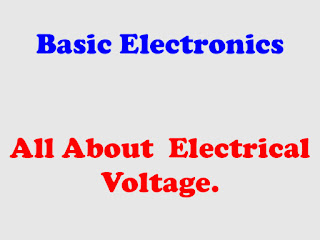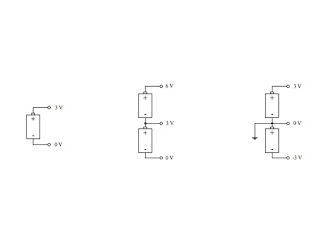Basic Electronics All About Electrical Voltage .Electrical Voltage

Electrical voltage :

Voltage When two charge distributions are separated by a distance, there exists an electrical force between the two. If the distributions are similar in charge (both positive or both negative), the force is opposing. If the charge distributions are of opposite charge (one positive and the other negative), the force is attractive. If the two charge distributions are fixed in place and a small positive unit of charge is placed within the system, the positive unit of charge will be influenced by both charge distributions. The unit of charge will move toward the negatively charged distribution (“pulled” by the negatively charged object and “pushed” by the positively charged object). An electrical field is used to describe the magnitude and direction of the force placed on the positive unit of charge due to the charge distributions. When the positive unit of charge moves from one point to another within this configuration, it will change in potential energy. This change in potential energy is equivalent to the work done by the positive unit of charge over a distance. Now, if we divide the potential energy by the positive unit of charge, we get what is called a voltage (or electrical potential—not to be confused with electrical potential energy). Often the terms potential and electromotive force (emf) are used instead of voltage.

Definition of voltage :

Voltage (symbolized V) is defined as the amount of energy required to move a unit of electrical charge from one place to another (potential energy/unit of charge). The unit for voltage is the volt (abbreviated with a V, which is the same as the symbol, so watch out). One volt is equal to one joule per coulomb:

1 V = 1 J/C

Streamline the concept of voltage :

In terms of electronics, it is often helpful to treat voltage as a kind of “electrical pressure” similar to that of water pressure. An analogy for this shown in (Fig 1) can be made between a tank filled with water and two sets of charged parallel plates.Figure 1

Objects with opposite charges attract, that is, they exert a force upon each other that
pulls them together. In this case, the magnitude of the force is proportional to the product of the charge on each mass. Like gravity, electrical force depends inversely on the
distance squared between the two bodies; the closer the bodies the greater the force.
Consequently, it takes energy to pull apart objects that are positively and negatively
charged, in the same manner that it takes energy to raise a big mass against the pull of
gravity.

The potential that separate objects with opposite charges have for doing work is
called voltage. Voltage is measured in units of volts (V). The unit is named for the
Italian scientist Alessandro Volta.

The greater the charge and the greater the separation, the greater the stored energy, or voltage. By the same token, the greater the voltage, the greater the force
that drives the charges together.

In the tank system, water pressure is greatest toward the bottom of the tank because of the weight from the water above. If a number of holes are drilled in the side of the tank, water will shoot out to escape the higher pressure inside. The further down the hole is drilled in the tank, the further out the water will shoot from it. The exiting beam of water will bend toward the ground due to gravity.

Now, if we take the water to be analogous to a supply of positively charged particles and take the water pressure to be analogous to the voltage across the plates in the electrical system, the positively charged particles will be drawn away from the positive plate (a) and move toward the negatively charged plate (b). The charges will be “escaping from the higher voltage to the lower voltage (analogous to the water escaping from the tank). As the charges move toward plate (b), the voltage across plates (c) and (d) will bend the beam of positive charges toward plate (d)—positive charges again are moving to a lower voltage. (This is analogous to the water beam bending as a result of the force of gravity as it escapes the tank.) The higher the voltage between plates (a) and (b), the less the beam of charge will be bent toward plate (d).

The ground :

Voltage is always measured between two points that represent the positive and
negative charges. In order to compare voltages of several charged bodies a common
reference point is necessary. This point is usually called "ground."

Understanding voltages becomes a relativity game. For example, to say a point in a circuit has a voltage of 10 V is meaningless unless you have another point in the circuit with which to compare it. Typically, the earth, with its infinite charge-absorbing ability and net zero charge, acts as a good point for comparison. It is considered the 0-V reference point or ground point. The symbol used for the ground is shown here:Figure 2

There are times when voltages are specified in circuits without reference to ground. For example, in (Fig 3), the first two battery systems to the left simply specify one battery terminal voltage with respect to another, while the third system to the right uses ground as a reference point.Figure 3

Measuring voltage :

(a) An arbitrary circuit diagram is shown as an illustration of how to use a voltmeter. Note that the meter measures the voltage drop across both the resistor and capacitor (which have identical voltage drops since they are connected in parallel). (b) A drawing of the same circuit showing how the leads for a DMM should be connected when measuring voltage. Notice how the meter is connected in parallel with the resistor.Figure 3